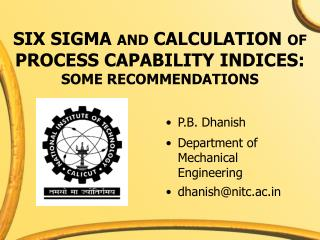DownloadDownload PresentationSIX SIGMA AND CALCULATION OF PROCESS CAPABILITY INDICES: SOME RECOMMENDATIONS

# SIX SIGMA AND CALCULATION OF PROCESS CAPABILITY INDICES: SOME RECOMMENDATIONS

Download Presentation## SIX SIGMA AND CALCULATION OF PROCESS CAPABILITY INDICES: SOME RECOMMENDATIONS

- - - - - - - - - - - - - - - - - - - - - - - - - - - E N D - - - - - - - - - - - - - - - - - - - - - - - - - - -
##### Presentation Transcript

1. SIX SIGMA AND CALCULATION OF PROCESS CAPABILITY INDICES: SOME RECOMMENDATIONS • P.B. Dhanish • Department of Mechanical Engineering • dhanish@nitc.ac.in

2. What is Six Sigma? The sample size problem The distribution problem The control problem Conclusion Overview

3. What is Six Sigma? • Disciplined quality improvement • AIM: Near elimination of defects • NUMERICALLY: 3.4 DPMO!

4. Defect levels at various sigmas

5. What is sigma? • Statistics: • The process standard deviation Then, six sigma? • Specification limits should be at • +/- six sigma

6. Then, how many defects? • Assuming Normal distribution • The area under the normal curve beyond +/-six sigma :Fraction non-conforming • Multiply by 1,000,000 to get DPMO • If the process is centred on target, 0.002 DPMO

7. Such perfect centering • Not possible in practice • Allow +/-1.5 sigma shift • Then the defect level will be 3.4DPMO

8. The sample size problem • In practice, true sigma is unknowable • Sample standard deviation • From a finite number of samples • Sampling error in sigma level • Single value not meaningful • Hence: Give Confidence Limits

9. If x is normally distributed • an upper 100(1-α)% confidence limit for σ is • and an upper 100(1- α)% confidence limit for μ is

10. Assuming that mean and sigma are independent • to calculate an upper 100(1-α)% confidence limit for the proportion nonconforming p, • construct 100(1-α)1/2 % confidence limits for each parameter separately • using these two values, determine p

11. For example, • If n=25, USL=6, LSL=-6, =0, and s=1, a 97.47% upper confidence limit for σ is and a 97.47% upper confidence limit for μ is

12. Then the proportion nonconforming: = 30.967ppm

13. Alternative: Determine sample size for the required confidence level

14. Recommendation 1 • Do NOT give a single value for the sigma level of your process • Instead, Give confidence limits OR the confidence level

15. The distribution problem • Above calculations utilise: • the tail end of the normal distribution • Does any process in nature match the values in the tail?

16. The values in the tail:

17. To check the correctness, • We need millions of samples! • No shift in the process during this production! • Hence impossible to verify • the exact values of the defect levels, say 3.4 or 5 DPMO, may not have practical significance

18. Not normally distributed: • Surface Finish • Circularity • Runout • Hence, take care

19. Cramer (1945): Lippman: • everybody believes in the law of errors, the experimenters because they think it is a mathematical theorem, the mathematicians because they think it is an experimental fact

20. Recommendation 2 • Verify that the distribution is normal • Otherwise, utilise the appropriate distribution • Realise that the exact values of low defect levels are meaningless

21. The Control Problem • To claim that future performance would be similar, the process should be stable OR in statistical control

22. Deming (1986): • One sees much wrong practice in connection with capability of the process. It is totally wrong to take any number of pieces such as 8, 20, 50 or 100, measure them with calipers or other instruments, and take 6 standard deviations of these measurements as the capability of the process

23. Consider thirty observations: -1.6, -1.2, -1.9, -0.6, -1.6, -1.4, -0.5, -0.9, -0.2, -0.7, 0.2, -0.5, 0.3, -0.4, 0.5, -0.3, 0.4, -0.2, 0.8, 0.6, 0, 1.2, 2, 0.5, 0.9, 0.8, 0.1, 1.4, 0.6, 1.7 • Mean 0 • Standard deviation 1

24. Histogram:

25. An excellent process? • If specification limits are +/-3 • Wait, Just plot a run chart for the given measurements

26. The process is drifting!

27. No capability can be ascribed! • QS9000: Distinguishes between Cpk and Ppk • An undisturbed process: Shouldn’t it be in control? • Very very unlikely • An SPC program necessary

28. Nelson (2001): • Getting a process in statistical control is not improvement (though it may be thought of as improvement of the operation), getting a process in statistical control only reveals the process, and after a process is in statistical control, improving it can begin.

29. Another pitfall: • Samples taken too infrequently • This way, any process can be made to appear in control! • A common cause for failure of SPC

30. Recommendation 3: • Ensure that the process is stable or in statistical control

31. Any questions?

32. Conclusion: Industries while claiming Six Sigma, should 1. reveal the confidence level of their sigma calculation 2. The process distribution utilised 3. How process stability was verified